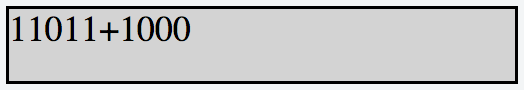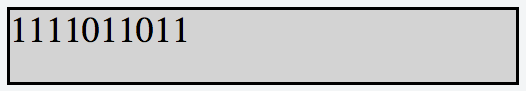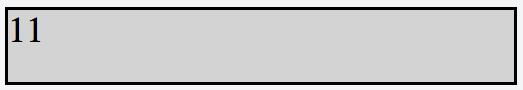## Algorithm

Hacker rank Problem Name: 10 Days of JavaScript - Day 9: Binary Calculator

Objective

In this challenge, we implement a calculator that uses binary numbers. Check out the attached tutorial for learning materials.

Implement a simple calculator that performs the following operations on binary numbers: addition, subtraction, multiplication, and division. Note that division operation must be integer division only; for example, 1001 / 100 = 10, 1110 / 101 = 10, and 101/1

The calculator's initial state must look like this:• Element IDs. Each element in the document must have an id, specified below:

innerHTML id Description/Behavior
res Contains the result of button presses.
btns A button container that displays all eight calculator buttons.
0 btn0 A button expressing binary digit
 . 1 btn1 A button expressing binary digit
 . C btnClr A button to clear the contents of

 . = btnEql A button to evaluate the contents of the expression in

•  . + btnSum A button for the addition operation. - btnSub A button for the subtraction operation. * btnMul A button for the multiplication operation. / btnDiv A button for the integer division operation.
• Styling. The document's elements must have the following styles:

• body has a width of 33%.
• res has a background-color of lightgray, a border that is solid, a height of 48px, and a font-size of 20px.
• btn0 and btn1 have a background-color of lightgreen and a color of brown.
• btnClr and btnEql have a background-color of darkgreen and a color of white.
• btnSum, btnSub, btnMul, and btnDiv have a background-color of black, a color of red.
• All the buttons in btns have a width of 25%, a height of 36px, a font-size of 18px, margin of 0px, and float value left.

The .js and .css files are in different directories, so use the link tag to provide the CSS file path and the script tag to provide the JS file path:

<!DOCTYPE html>
<html>

<body>
<script src="js/binaryCalculator.js" type="text/javascript"></script>
</body>
</html>


Constraints

• All expressions in the test dataset are entered in the formoperand1 -> operator -> operand2, where operand1 is the first binary number,operand2 is the second binary number, and operator is in the set {+, -, *, =}
• Both operands will always be positive integers when converted from base- 2 to base- 10
• All expressions will be valid.

Explanation

Consider the following sequence of button clicks:

1 -> 1 -> 0 -> 1-> 1 -> + -> 1 -> 1 -> 0 ->-> 0 -> 0 ->=

Before pressing the = button, the result div looks like this:After pressing the = button to evaluate our expression, the result div looks like this:Notice that (11011)2 = (27)10, (1000)2 = (8)10, and (100011)2 = (35)10, so our calculator evaluated the expression correctly.

Now, let's consider our next sequence of button clicks as:

0 -> 1 -> * -> 1 -> 1 -> 1 ->=

Before pressing the = button, the result div looks like this:After pressing the = button to evaluate our expression, the result div looks like thisonsider the next sequence of button clicks as:

C -> 1 -> 1

The result div looks like this:## Code Examples

### #1 Code Example with Javascript Programming

Code - Javascript Programming


var resultScreen=document.getElementById("res");
var result=0;
function clickZero()
{

resultScreen.innerHTML+="0";
}
function clickOne()
{

resultScreen.innerHTML+="1";
}
function clickSum()
{
operatorSeq="+";
result=parseInt(resultScreen.innerHTML,2);
resultScreen.innerHTML+="+";
}
function clickSub()
{
operatorSeq="-";
resultScreen.innerHTML+="-";
}
function clickMul()
{
operatorSeq="*";
result=parseInt(resultScreen.innerHTML,2);
resultScreen.innerHTML+="*";
}
function clickDiv()
{
operatorSeq="/";
resultScreen.innerHTML+="/";
}
function clickEql()
{
var ans=0;

if(operatorSeq=='+')
{
var i =(resultScreen.innerHTML).indexOf("+");

var operand2=parseInt((resultScreen.innerHTML).substr(i+1),2);
ans =result+operand2;
}
else if(operatorSeq=='-')

{
var i =(resultScreen.innerHTML).indexOf("-");
var operand2=parseInt((resultScreen.innerHTML).substr(i+1),2);
ans =result-operand2;
}

else if(operatorSeq=='*')

{
var i =(resultScreen.innerHTML).indexOf("*");
var operand2=parseInt((resultScreen.innerHTML).substr(i+1),2);
ans =result*operand2;
}
else if(operatorSeq=='/')

{
var i =(resultScreen.innerHTML).indexOf("/");
var operand2=parseInt((resultScreen.innerHTML).substr(i+1),2);
ans =result/operand2;
}

resultScreen.innerHTML=ans.toString(2);
}
function clickClear()
{
resultScreen.innerHTML="";

}

Copy The Code &